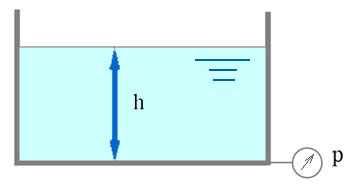# Calculation of pressure at a depth of 1, 5, 10, 100 meters

This calculator determines the gauge pressure in relation to the depth of the liquid. You can enter the depth and density of the liquid and calculate the pressure.

This calculator does not add air pressure at the surface - the results are relative to the surface pressure.

The result of the calculation is the gauge pressure. The gauge pressure is zero-referenced against atmospheric pressure.

## Online calculator. Pressure at depth calculator

Choose a liquid. Specify the depth.

### Initial data

Depth h:
Liquid:
water
sea water
mineral oil
petrol
machine oil
mercury
density of another liquid kg/m3

## Calculation of pressure at a depth

A pressure at depth is given by::

P=ρ × g × h
• ρ - density of a liquid
• h - depth
• g - acceleration of gravity
• P - pressureLanguages
Spanish (ES) Deutsch (DE)
Featured articles
How to read hydraulic circuits
Hydraulic cylinders
Hydraulic accumulators
Best calculators
Pressure loss calculator
Calculating the system curve
Hydraulic cylinder force calculator
My Youtube channel
This site uses cookies to store information on your computer. Details